# Oxidation Reduction Reactions Redox TerminologyREVIEW CHAPTER 4 redox

• Slides: 44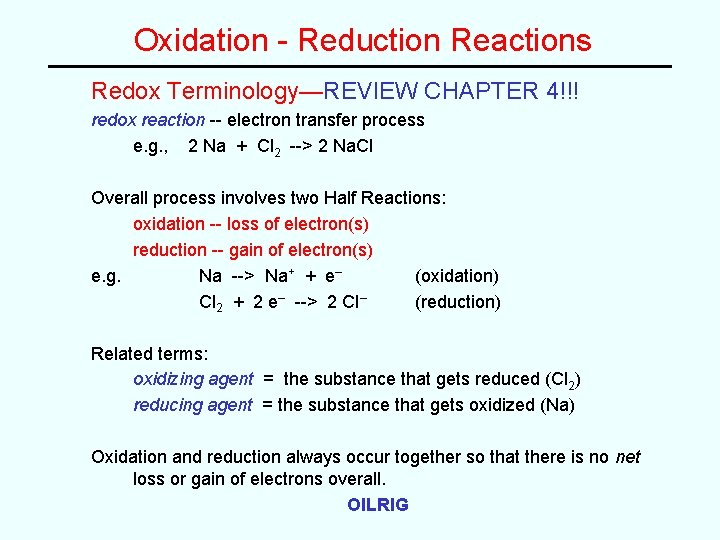Oxidation - Reduction Reactions Redox Terminology—REVIEW CHAPTER 4!!! redox reaction -- electron transfer process e. g. , 2 Na + Cl 2 --> 2 Na. Cl Overall process involves two Half Reactions: oxidation -- loss of electron(s) reduction -- gain of electron(s) e. g. Na --> Na+ + e– (oxidation) Cl 2 + 2 e– --> 2 Cl– (reduction) Related terms: oxidizing agent = the substance that gets reduced (Cl 2) reducing agent = the substance that gets oxidized (Na) Oxidation and reduction always occur together so that there is no net loss or gain of electrons overall. OILRIGRedox Reactions • The transfer of electrons between species, meaning that one species is oxidized and one is reduced. The two processes will always occur together. • When has a redox reaction occurred? – If there is a change in the oxidation state of any element in the reaction, a redox reaction has happened. – Remember that if something is oxidized, something else must be reduced! (and vice-versa) More examples: 2 Ca(s) + O 2(g) --> 2 Ca. O(s) 2 Al(s) + 3 Cl 2(g) --> 2 Al. Cl 3(s)HALF-REACTION METHOD for balancing Redox Equations 1. Write unbalanced ionic equations for the two half-reactions. (Look at oxidation numbers in the “skeleton equation. ”) 2. Balance atoms other than H and O. 3. Add appropriate number of electrons. 4. Balance O with H 2 O. 5. Balance H with H+. 6. If in acidic solution, then skip to step 7. If in basic solution, then add equal number of OH– to both sides to cancel all of the H+. 7. Multiply balanced half-reactions by appropriate coefficients so that the number of electrons are equal. 8. Rewrite the balanced half reactions. 9. Add the half-reactions together. 10. Cancel species that appear on both sides to get the balanced Net Ionic Equation. 11. If necessary, add spectator ions to get the balanced molecular equation. Check the Final Balance (atoms and charges)!Example Problem Balance the following redox equation. Ce 4+(aq) + Sn 2+(aq) --> Ce 3+(aq) + Sn 4+(aq) The reaction can be separated into a reaction involving the substance being reduced; Ce 4+(aq) + e– --> Ce 3+(aq) And the substance being oxidized; Sn 2+(aq) --> Sn 4+(aq) + 2 e– We can see that the equations don’t balance; you must multiply the top equation by two, then add them to get 2 Ce 4+(aq) + Sn 2+(aq) --> 2 Ce 3+(aq) + Sn 4+(aq)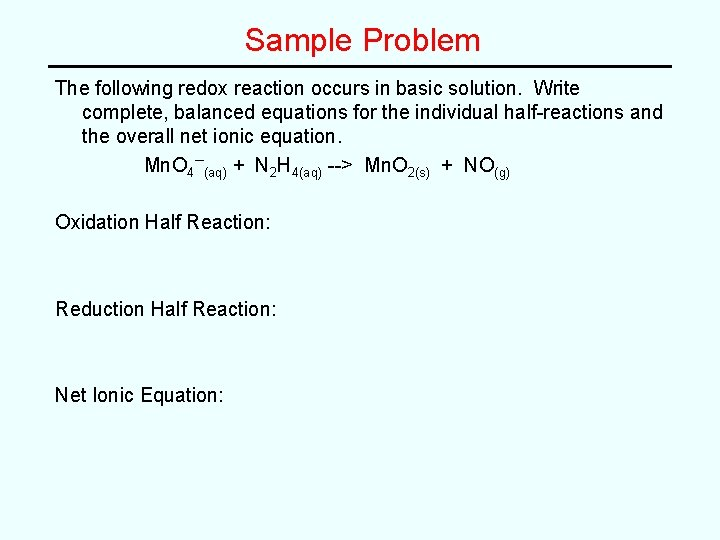Sample Problem The following redox reaction occurs in basic solution. Write complete, balanced equations for the individual half-reactions and the overall net ionic equation. Mn. O 4–(aq) + N 2 H 4(aq) --> Mn. O 2(s) + NO(g) Oxidation Half Reaction: Reduction Half Reaction: Net Ionic Equation: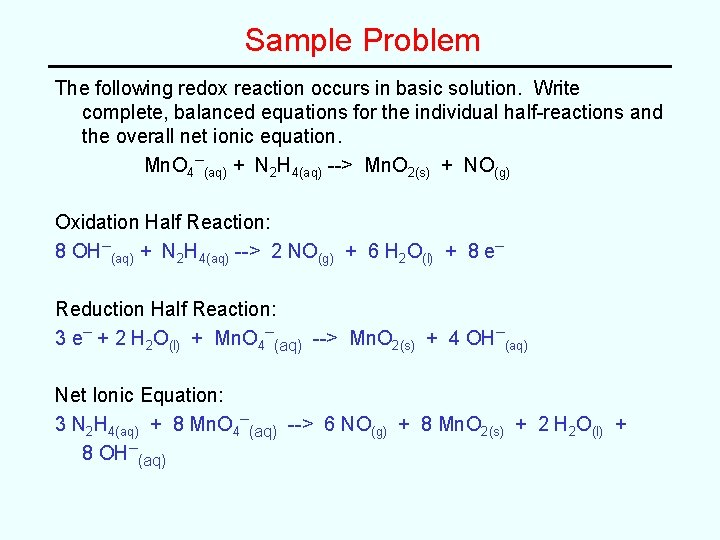Sample Problem The following redox reaction occurs in basic solution. Write complete, balanced equations for the individual half-reactions and the overall net ionic equation. Mn. O 4–(aq) + N 2 H 4(aq) --> Mn. O 2(s) + NO(g) Oxidation Half Reaction: 8 OH–(aq) + N 2 H 4(aq) --> 2 NO(g) + 6 H 2 O(l) + 8 e– Reduction Half Reaction: 3 e– + 2 H 2 O(l) + Mn. O 4–(aq) --> Mn. O 2(s) + 4 OH–(aq) Net Ionic Equation: 3 N 2 H 4(aq) + 8 Mn. O 4–(aq) --> 6 NO(g) + 8 Mn. O 2(s) + 2 H 2 O(l) + 8 OH–(aq)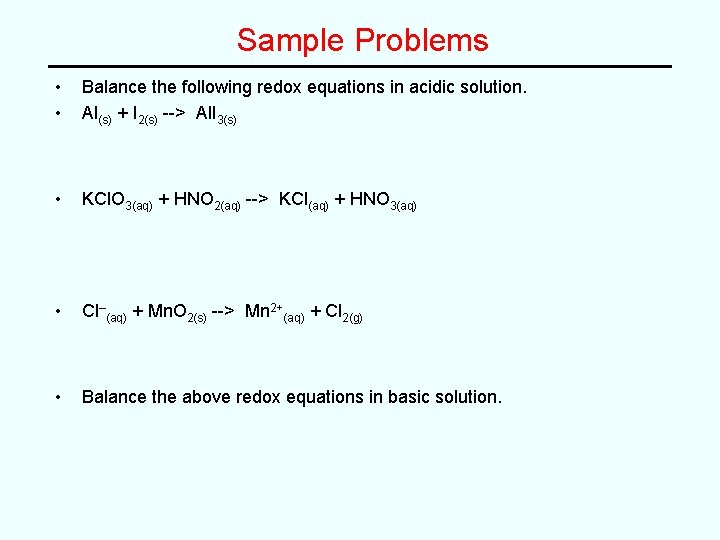Sample Problems • • Balance the following redox equations in acidic solution. Al(s) + I 2(s) --> Al. I 3(s) • KCl. O 3(aq) + HNO 2(aq) --> KCl(aq) + HNO 3(aq) • Cl–(aq) + Mn. O 2(s) --> Mn 2+(aq) + Cl 2(g) • Balance the above redox equations in basic solution.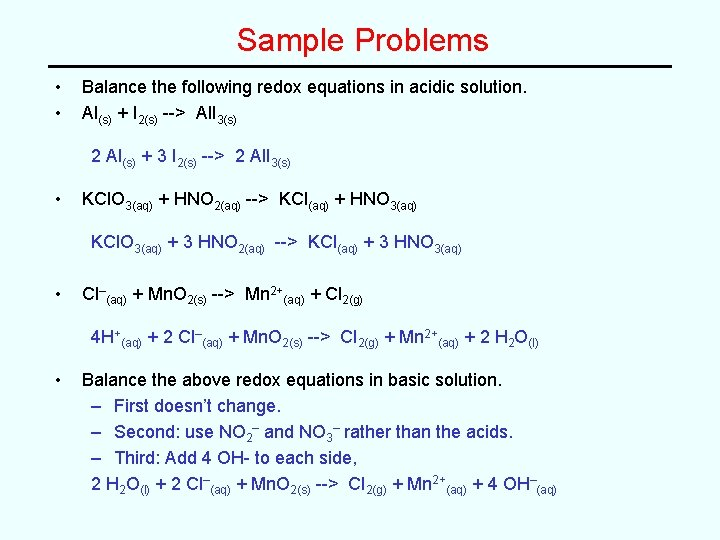Sample Problems • • Balance the following redox equations in acidic solution. Al(s) + I 2(s) --> Al. I 3(s) 2 Al(s) + 3 I 2(s) --> 2 Al. I 3(s) • KCl. O 3(aq) + HNO 2(aq) --> KCl(aq) + HNO 3(aq) KCl. O 3(aq) + 3 HNO 2(aq) --> KCl(aq) + 3 HNO 3(aq) • Cl–(aq) + Mn. O 2(s) --> Mn 2+(aq) + Cl 2(g) 4 H+(aq) + 2 Cl–(aq) + Mn. O 2(s) --> Cl 2(g) + Mn 2+(aq) + 2 H 2 O(l) • Balance the above redox equations in basic solution. – First doesn’t change. – Second: use NO 2– and NO 3– rather than the acids. – Third: Add 4 OH- to each side, 2 H 2 O(l) + 2 Cl–(aq) + Mn. O 2(s) --> Cl 2(g) + Mn 2+(aq) + 4 OH–(aq)Electrochemistry Terminology Electrochemical Cell -a device that converts electrical energy into chemical energy or vice versa • Two Types: Electrolytic cell Converts electrical energy into chemical energy Electricity is used to drive a non-spontaneous reaction Galvanic (or voltaic) cell Converts chemical energy into electricity (a battery!) A spontaneous reaction produces electricity • Conduction: Metals: Solutions: (or molten salts) metallic (electronic) conduction -- free movement of electrons electrolytic (ionic) conduction -- free movement of ions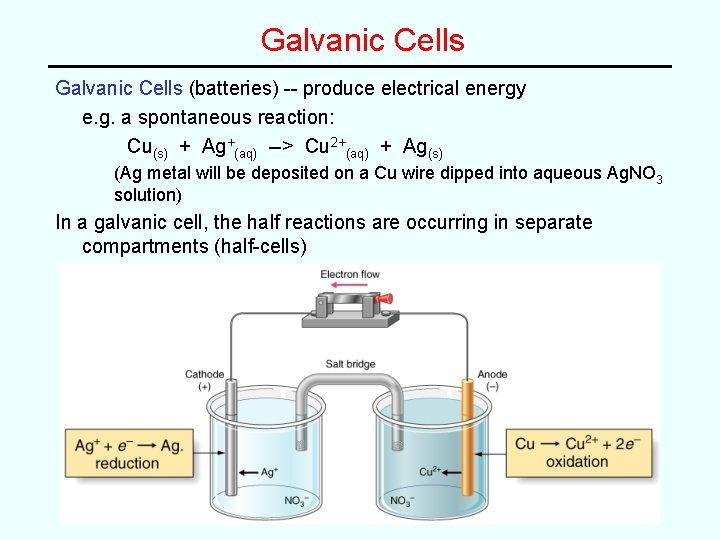Galvanic Cells (batteries) -- produce electrical energy e. g. a spontaneous reaction: Cu(s) + Ag+(aq) --> Cu 2+(aq) + Ag(s) (Ag metal will be deposited on a Cu wire dipped into aqueous Ag. NO 3 solution) In a galvanic cell, the half reactions are occurring in separate compartments (half-cells)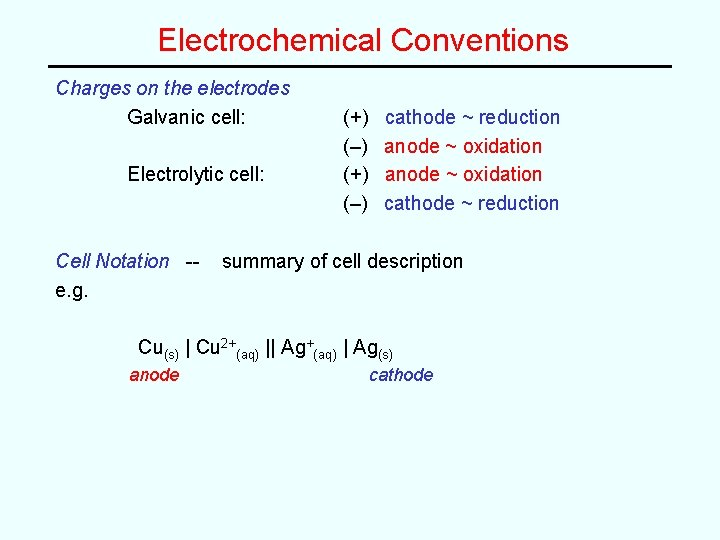Electrochemical Conventions Charges on the electrodes Galvanic cell: Electrolytic cell: Cell Notation -e. g. (+) (–) cathode ~ reduction anode ~ oxidation cathode ~ reduction summary of cell description Cu(s) | Cu 2+(aq) || Ag+(aq) | Ag(s) anode cathodeCell Potential Cell potential ~ Eºcell (an electromotive force, emf) Units of Eºcell are volts: 1 volt = 1 joule/coulomb Eºcell is a measure of the relative spontaneity of a cell reaction Positive (+) Eºcell --> spontaneous reaction Eºcell depends on: – Nature of reactants – Temperature -superscript º means 25 ºC – Concentrations -- superscript º means all conc are at 1. 00 M and gases are at 1. 00 atm but, Eºcell is independent of amounts of reactants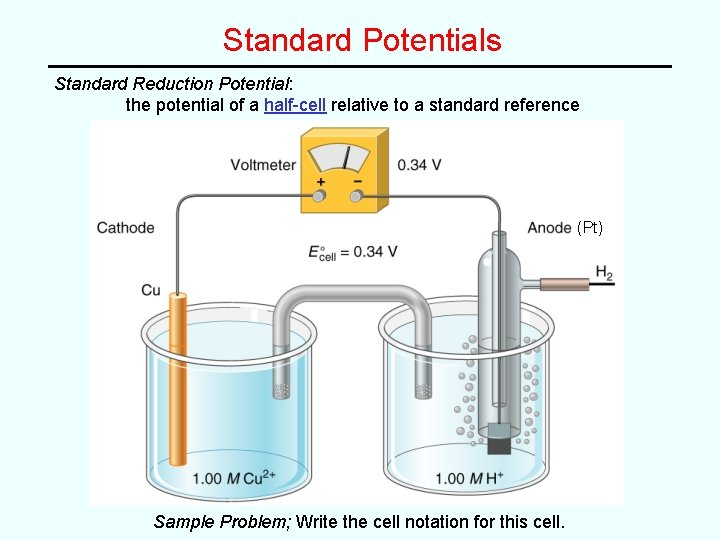Standard Potentials Standard Reduction Potential: the potential of a half-cell relative to a standard reference (Pt) Sample Problem; Write the cell notation for this cell.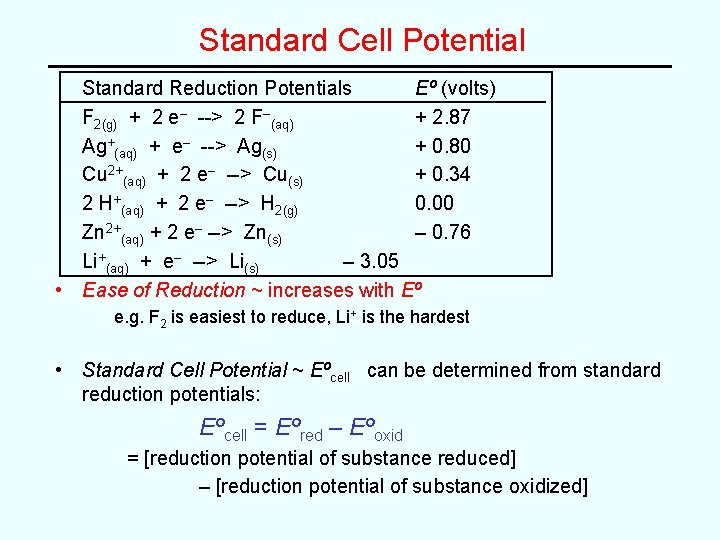Standard Cell Potential Standard Reduction Potentials Eº (volts) F 2(g) + 2 e– --> 2 F–(aq) + 2. 87 Ag+(aq) + e– --> Ag(s) + 0. 80 Cu 2+(aq) + 2 e– --> Cu(s) + 0. 34 2 H+(aq) + 2 e– --> H 2(g) 0. 00 Zn 2+(aq) + 2 e– --> Zn(s) – 0. 76 Li+(aq) + e– --> Li(s) – 3. 05 • Ease of Reduction ~ increases with Eº e. g. F 2 is easiest to reduce, Li+ is the hardest • Standard Cell Potential ~ Eºcell can be determined from standard reduction potentials: Eºcell = Eºred – Eºoxid = [reduction potential of substance reduced] – [reduction potential of substance oxidized]Standard Potential Example Problem Is the following a galvanic or an electrolytic cell? Write the balanced cell reaction and calculate Eºcell. Zn(s) | Zn 2+(aq) || Cu 2+(aq) | Cu(s) Cathode: Cu 2+ + 2 e– --> Cu Eº = 0. 34 V Anode: Zn --> Zn 2+ + 2 e– Eº = – 0. 76 V Cell rxn: Zn(s) + Cu 2+(aq) --> Zn 2+(aq) + Cu(s) Eºcell = Eºred – Eºox = 0. 34 V – (– 0. 76 V) = + 1. 10 V ∴ galvanic.Another Example Problem Given the standard reduction potentials: O 2(g) + 4 H+(aq) + 4 e– --> 2 H 2 O(l) Eº = 1. 23 V Cl 2(g) + 2 e– --> 2 Cl–(aq) Eº = 1. 36 V Write a balanced equation and calculate Eºcell for a galvanic cell based on these half reactions. Sketch the galvanic cell. “galvanic” implies a positive value for Eºcell so, Cl 2/Cl– should be the reduction half reaction, since 1. 36 – 1. 23 = + 0. 13 V ∴ reverse the O 2 half reaction and make it the oxidation Multiply the Cl 2 reaction by 2, to make e–’s cancel, hence: 2 Cl 2(g) + 2 H 2 O(l) --> 4 Cl–(aq) + O 2(g) + 4 H+(aq) Note: When a half-reaction is multiplied by a coefficient, the Eº IS NOT MULTIPLIED by the coefficient.Diagram of cell e– e– voltmeter Pt electrode salt bridge cathode (reduction) O 2 gas Cl 2 gas K+ K+ Cl– NO 3– K+ K+ NO 3– supporting electrolyte NO 3– anode (oxidation)Sample Problem Under standard conditions, is the following a galvanic or an electrolytic cell? Support your conclusion with appropriate calculations and balanced chemical reactions. Ag(s), Ag. Br(s) | Br–(aq) || Au 3+(aq) | Au(s) --From table, Au 3+(aq) + 3 e – Au(s) Eº = 1. 50 V Ag. Br(s) + e – Ag(s) + Br –(aq) Eº = 0. 071 V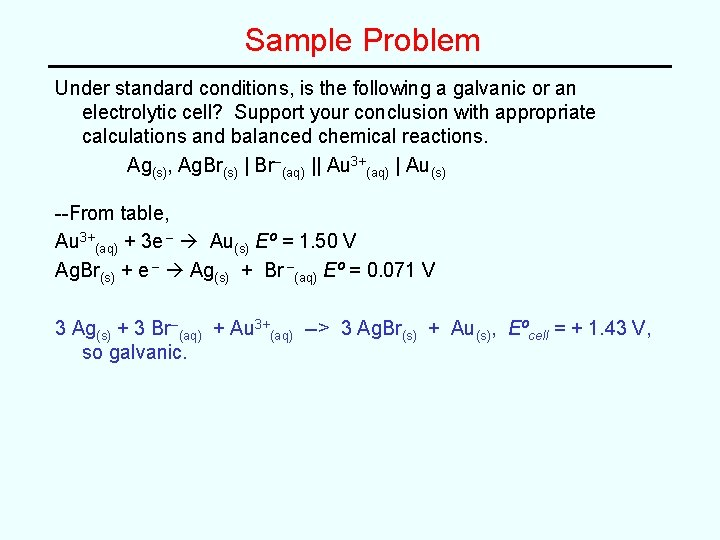Sample Problem Under standard conditions, is the following a galvanic or an electrolytic cell? Support your conclusion with appropriate calculations and balanced chemical reactions. Ag(s), Ag. Br(s) | Br–(aq) || Au 3+(aq) | Au(s) --From table, Au 3+(aq) + 3 e – Au(s) Eº = 1. 50 V Ag. Br(s) + e – Ag(s) + Br –(aq) Eº = 0. 071 V 3 Ag(s) + 3 Br–(aq) + Au 3+(aq) --> 3 Ag. Br(s) + Au(s), Eºcell = + 1. 43 V, so galvanic.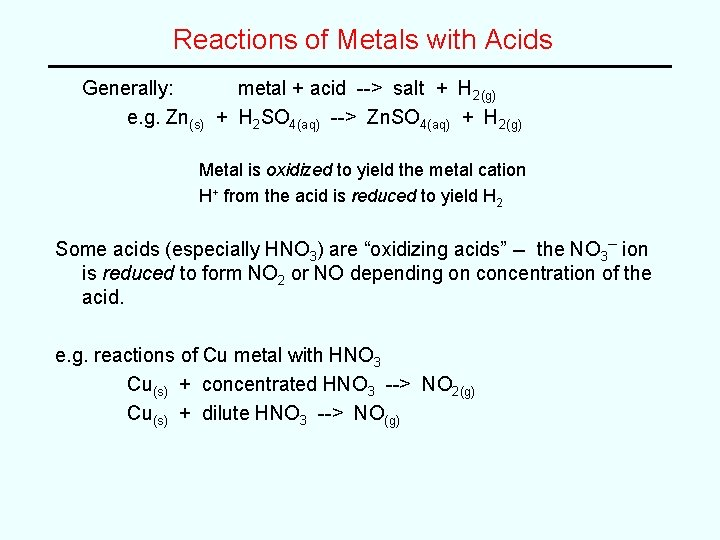Reactions of Metals with Acids Generally: metal + acid --> salt + H 2(g) e. g. Zn(s) + H 2 SO 4(aq) --> Zn. SO 4(aq) + H 2(g) Metal is oxidized to yield the metal cation H+ from the acid is reduced to yield H 2 Some acids (especially HNO 3) are “oxidizing acids” -- the NO 3– ion is reduced to form NO 2 or NO depending on concentration of the acid. e. g. reactions of Cu metal with HNO 3 Cu(s) + concentrated HNO 3 --> NO 2(g) Cu(s) + dilute HNO 3 --> NO(g)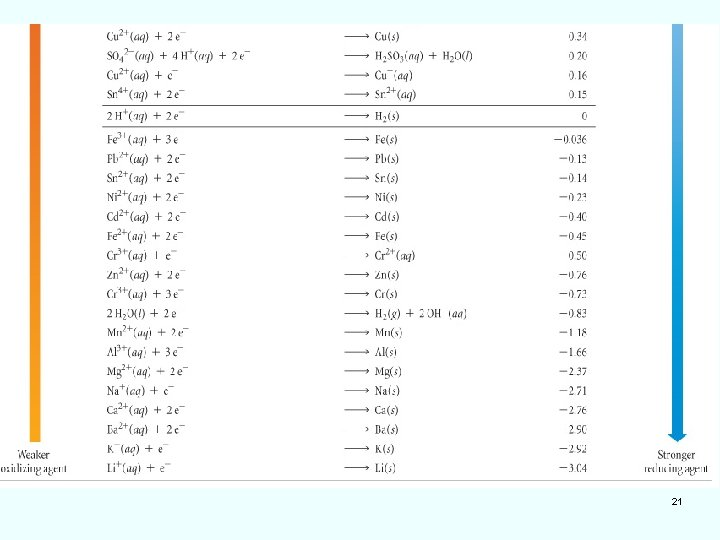21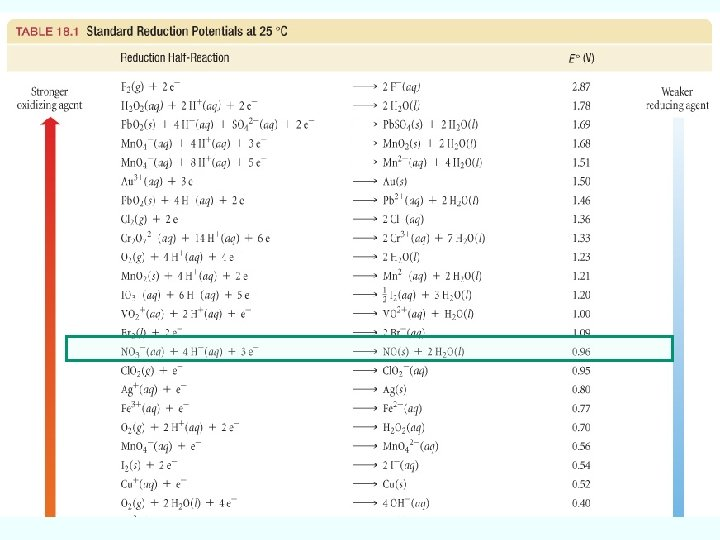Cell Potential and Thermodynamics Free Energy Change (DG) DG = – Wmax (maximum work) • For an electrochemical cell: W = n. FEcell where n = # moles e– F = 96, 500 coulombs/mole e– joule Units: volt = coulombs joules = (moles e–) mole e– joule coulomb ∴ free energy is related to Ecell as follows: DG = – n. FEcell under standard conditions: use DGº and EºcellEquilibrium Constant since DGº = – RTln. Kc = – n. FEºcell can rearrange to: RT Eºcell = n. F ln Kc (Use 8. 314 J mol-1 K-1 for R!) at 25 ºC, use values for R, T, and F, then convert to base-10 log: 0. 0592 Eºcell = n Overall, log Kc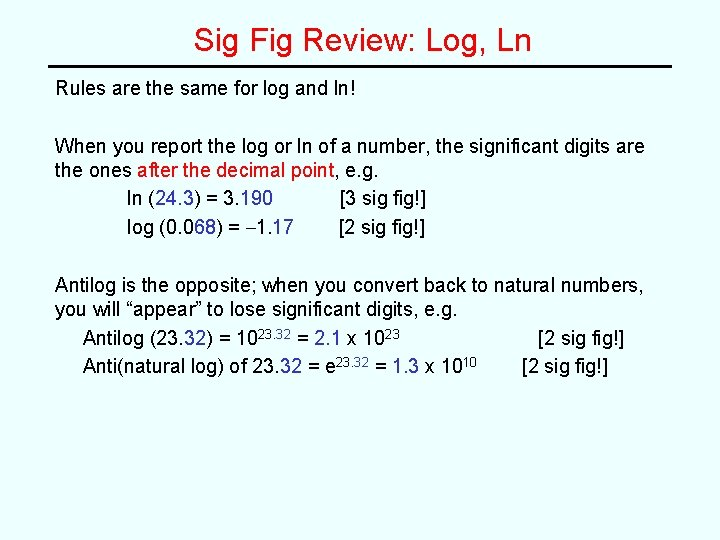Sig Fig Review: Log, Ln Rules are the same for log and ln! When you report the log or ln of a number, the significant digits are the ones after the decimal point, e. g. ln (24. 3) = 3. 190 [3 sig fig!] log (0. 068) = – 1. 17 [2 sig fig!] Antilog is the opposite; when you convert back to natural numbers, you will “appear” to lose significant digits, e. g. Antilog (23. 32) = 1023. 32 = 2. 1 x 1023 [2 sig fig!] Anti(natural log) of 23. 32 = e 23. 32 = 1. 3 x 1010 [2 sig fig!]Sample Problem Given the following standard reduction potentials, calculate the solubility product constant (Ksp) for lead sulfate, Pb. SO 4(s) + 2 e– --> Pb(s) + SO 42–(aq) Eº = – 0. 360 V Pb 2+(aq) + 2 e– --> Pb(s) Eº = – 0. 130 V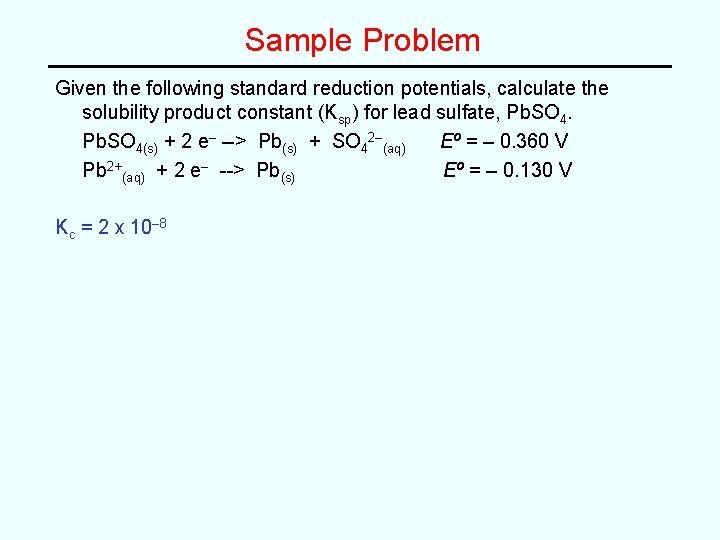Sample Problem Given the following standard reduction potentials, calculate the solubility product constant (Ksp) for lead sulfate, Pb. SO 4(s) + 2 e– --> Pb(s) + SO 42–(aq) Eº = – 0. 360 V Pb 2+(aq) + 2 e– --> Pb(s) Eº = – 0. 130 V Kc = 2 x 10– 8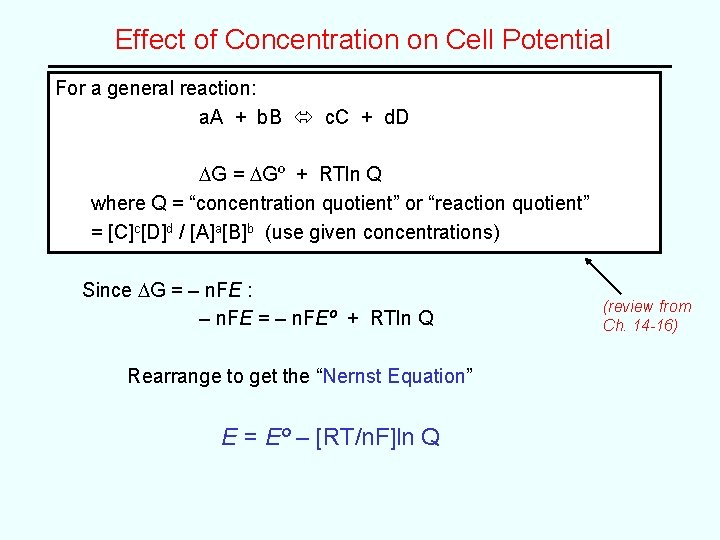Effect of Concentration on Cell Potential For a general reaction: a. A + b. B c. C + d. D DG = DGº + RTln Q where Q = “concentration quotient” or “reaction quotient” = [C]c[D]d / [A]a[B]b (use given concentrations) Since DG = – n. FE : – n. FE = – n. FEº + RTln Q Rearrange to get the “Nernst Equation” E = Eº – [RT/n. F]ln Q (review from Ch. 14 -16)The Nernst Equation E = Eº – [RT/n. F]ln Q The Nernst Equation shows the relationship between the standard cell potential (Eº) and the cell potential (E) under actual, non-standard conditions. this can be simplified at 25 ºC to: E = Eº – (0. 0592/n)log Q Major use of the Nernst Equation: • Determine concentrations from standard reduction potentials • Use actual concentrations (i. e. Q) to calculate Ecell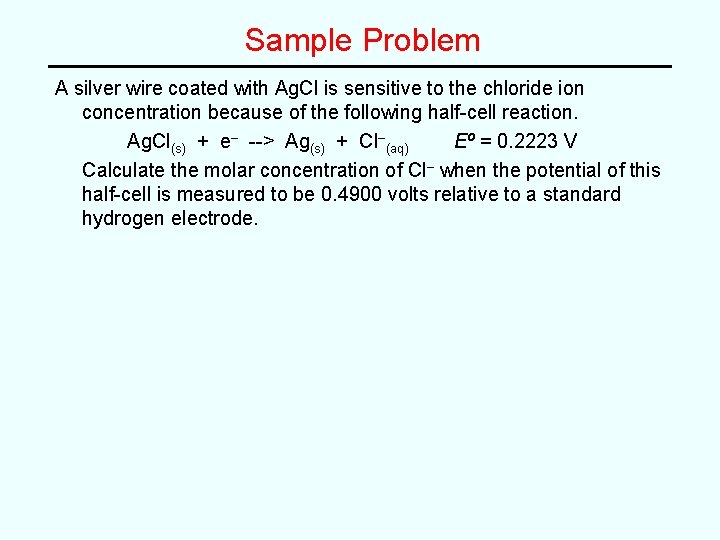Sample Problem A silver wire coated with Ag. Cl is sensitive to the chloride ion concentration because of the following half-cell reaction. Ag. Cl(s) + e– --> Ag(s) + Cl–(aq) Eº = 0. 2223 V Calculate the molar concentration of Cl– when the potential of this half-cell is measured to be 0. 4900 volts relative to a standard hydrogen electrode.Sample Problem A silver wire coated with Ag. Cl is sensitive to the chloride ion concentration because of the following half-cell reaction. Ag. Cl(s) + e– --> Ag(s) + Cl–(aq) Eº = 0. 2223 V Calculate the molar concentration of Cl– when the potential of this half-cell is measured to be 0. 4900 volts relative to a standard hydrogen electrode. 3 x 10– 5 M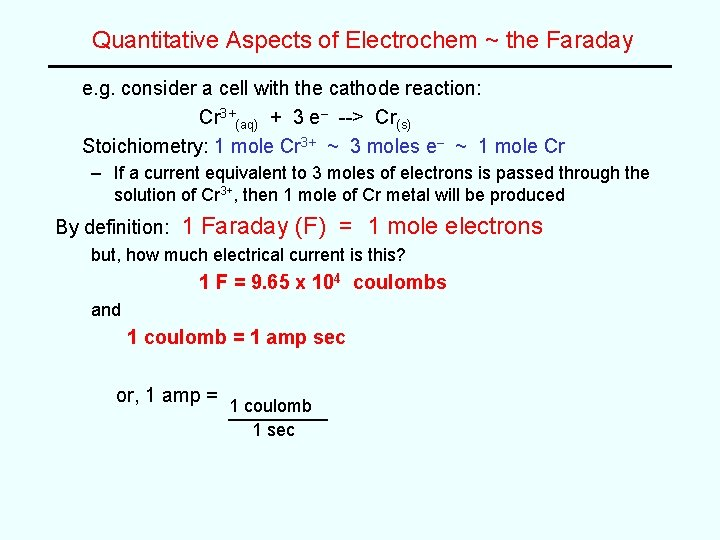Quantitative Aspects of Electrochem ~ the Faraday e. g. consider a cell with the cathode reaction: Cr 3+(aq) + 3 e– --> Cr(s) Stoichiometry: 1 mole Cr 3+ ~ 3 moles e– ~ 1 mole Cr – If a current equivalent to 3 moles of electrons is passed through the solution of Cr 3+, then 1 mole of Cr metal will be produced By definition: 1 Faraday (F) = 1 mole electrons but, how much electrical current is this? 1 F = 9. 65 x 104 coulombs and 1 coulomb = 1 amp sec or, 1 amp = 1 coulomb 1 sec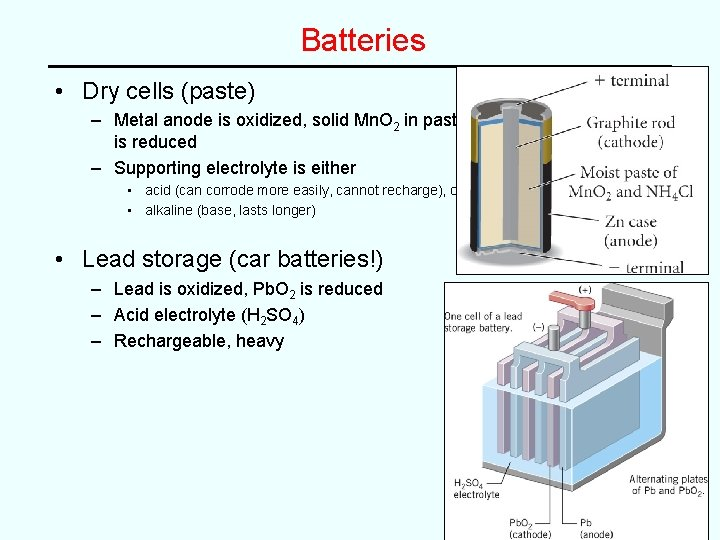Batteries • Dry cells (paste) – Metal anode is oxidized, solid Mn. O 2 in paste is reduced – Supporting electrolyte is either • acid (can corrode more easily, cannot recharge), or • alkaline (base, lasts longer) • Lead storage (car batteries!) – Lead is oxidized, Pb. O 2 is reduced – Acid electrolyte (H 2 SO 4) – Rechargeable, heavy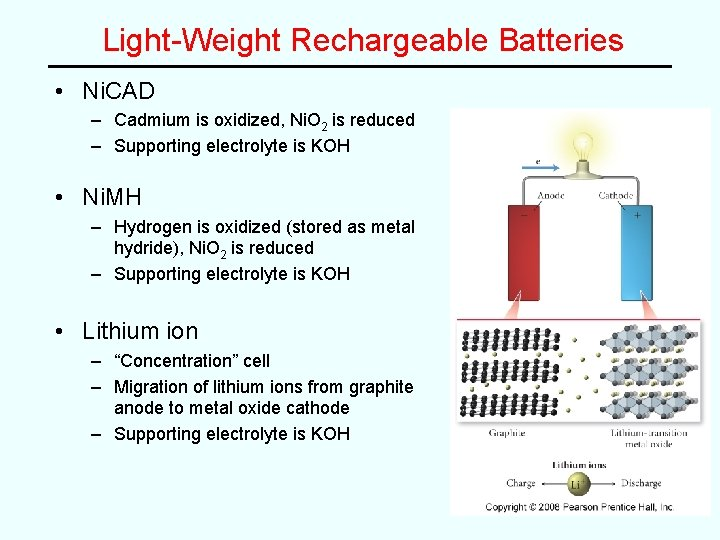Light-Weight Rechargeable Batteries • Ni. CAD – Cadmium is oxidized, Ni. O 2 is reduced – Supporting electrolyte is KOH • Ni. MH – Hydrogen is oxidized (stored as metal hydride), Ni. O 2 is reduced – Supporting electrolyte is KOH • Lithium ion – “Concentration” cell – Migration of lithium ions from graphite anode to metal oxide cathode – Supporting electrolyte is KOH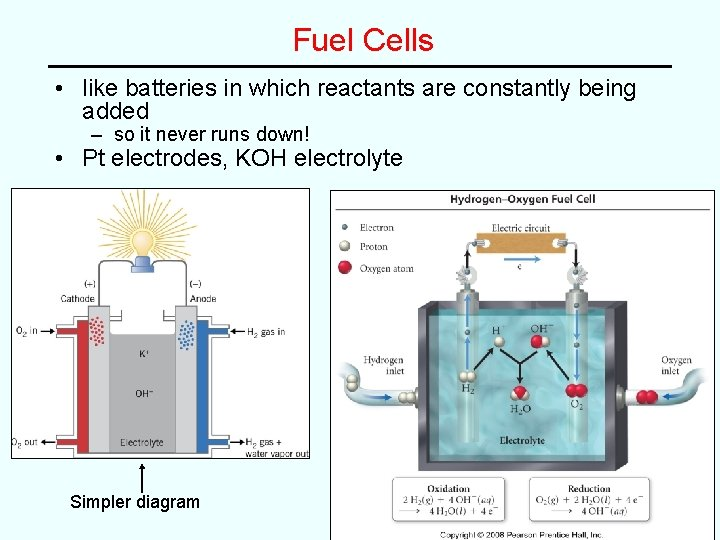Fuel Cells • like batteries in which reactants are constantly being added – so it never runs down! • Pt electrodes, KOH electrolyte Simpler diagram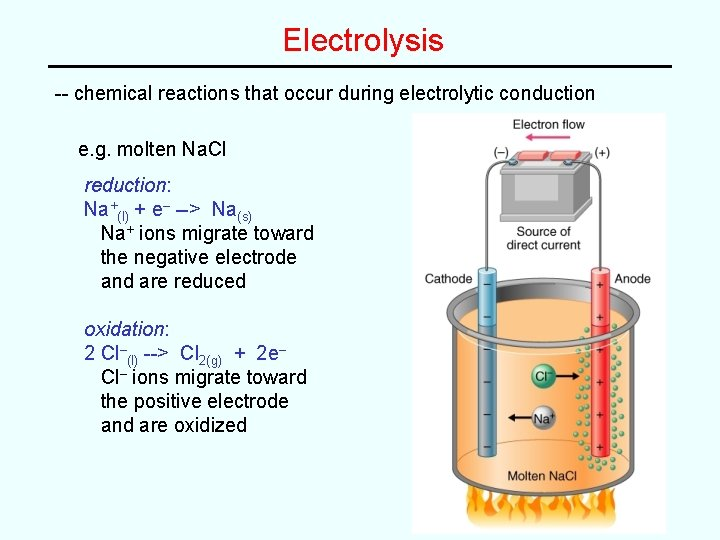Electrolysis -- chemical reactions that occur during electrolytic conduction e. g. molten Na. Cl reduction: Na+(l) + e– --> Na(s) Na+ ions migrate toward the negative electrode and are reduced oxidation: 2 Cl–(l) --> Cl 2(g) + 2 e– Cl– ions migrate toward the positive electrode and are oxidized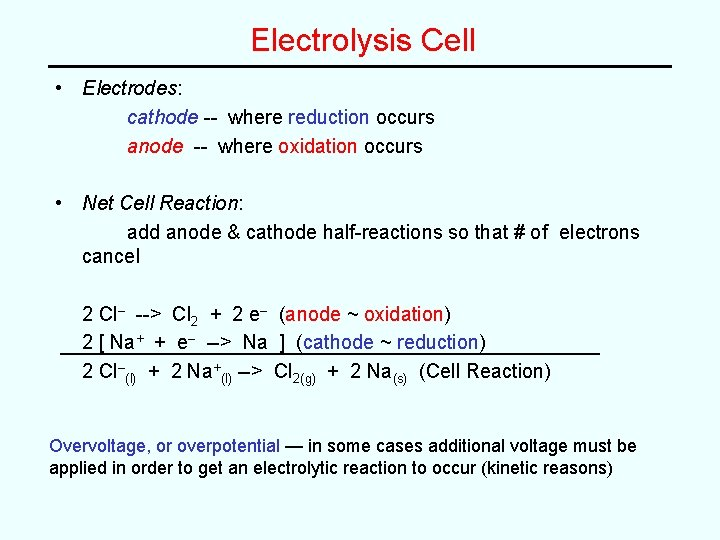Electrolysis Cell • Electrodes: cathode -- where reduction occurs anode -- where oxidation occurs • Net Cell Reaction: add anode & cathode half-reactions so that # of electrons cancel 2 Cl– --> Cl 2 + 2 e– (anode ~ oxidation) 2 [ Na+ + e– --> Na ] (cathode ~ reduction) 2 Cl–(l) + 2 Na+(l) --> Cl 2(g) + 2 Na(s) (Cell Reaction) Overvoltage, or overpotential — in some cases additional voltage must be applied in order to get an electrolytic reaction to occur (kinetic reasons)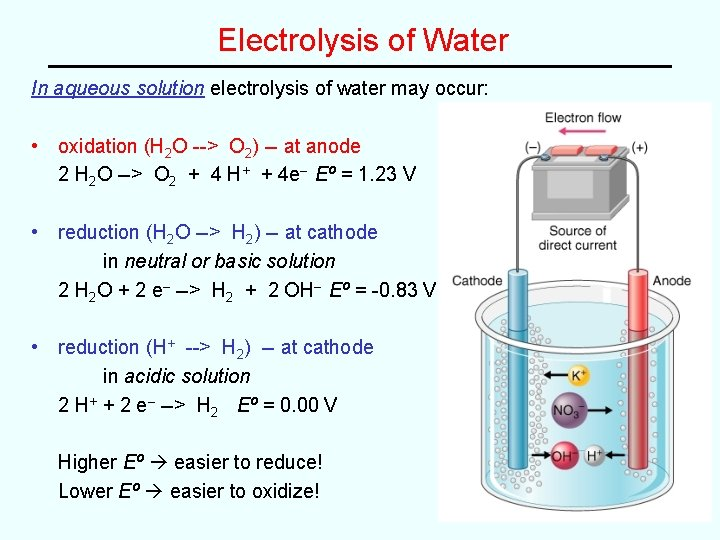Electrolysis of Water In aqueous solution electrolysis of water may occur: • oxidation (H 2 O --> O 2) -- at anode 2 H 2 O --> O 2 + 4 H+ + 4 e– Eº = 1. 23 V • reduction (H 2 O --> H 2) -- at cathode in neutral or basic solution 2 H 2 O + 2 e– --> H 2 + 2 OH– Eº = -0. 83 V • reduction (H+ --> H 2) -- at cathode in acidic solution 2 H+ + 2 e– --> H 2 Eº = 0. 00 V Higher Eº easier to reduce! Lower Eº easier to oxidize!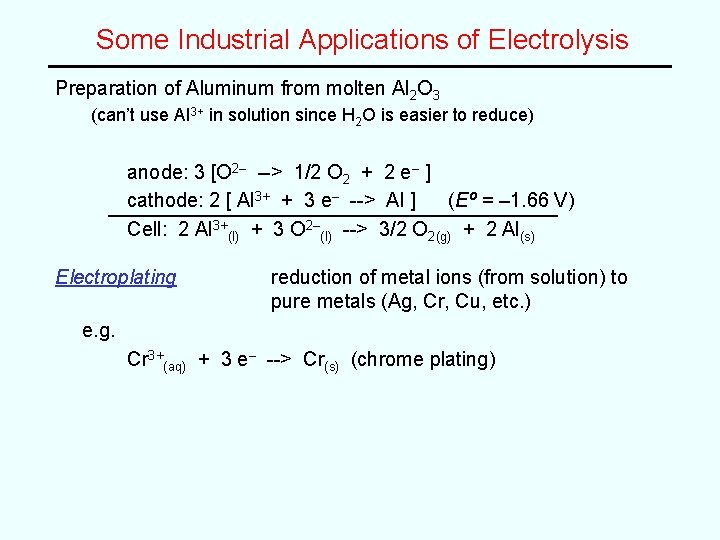Some Industrial Applications of Electrolysis Preparation of Aluminum from molten Al 2 O 3 (can’t use Al 3+ in solution since H 2 O is easier to reduce) anode: 3 [O 2– --> 1/2 O 2 + 2 e– ] cathode: 2 [ Al 3+ + 3 e– --> Al ] (Eº = – 1. 66 V) Cell: 2 Al 3+(l) + 3 O 2–(l) --> 3/2 O 2(g) + 2 Al(s) Electroplating reduction of metal ions (from solution) to pure metals (Ag, Cr, Cu, etc. ) e. g. Cr 3+(aq) + 3 e– --> Cr(s) (chrome plating)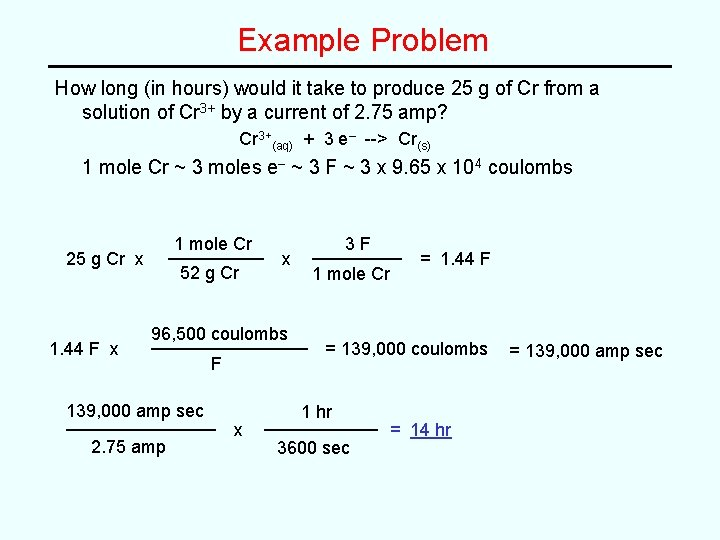Example Problem How long (in hours) would it take to produce 25 g of Cr from a solution of Cr 3+ by a current of 2. 75 amp? Cr 3+(aq) + 3 e– --> Cr(s) 1 mole Cr ~ 3 moles e– ~ 3 F ~ 3 x 9. 65 x 104 coulombs 1 mole Cr 25 g Cr x 1. 44 F x 52 g Cr x 96, 500 coulombs 139, 000 amp sec 2. 75 amp F x 3 F 1 mole Cr = 1. 44 F = 139, 000 coulombs 1 hr 3600 sec = 14 hr = 139, 000 amp sec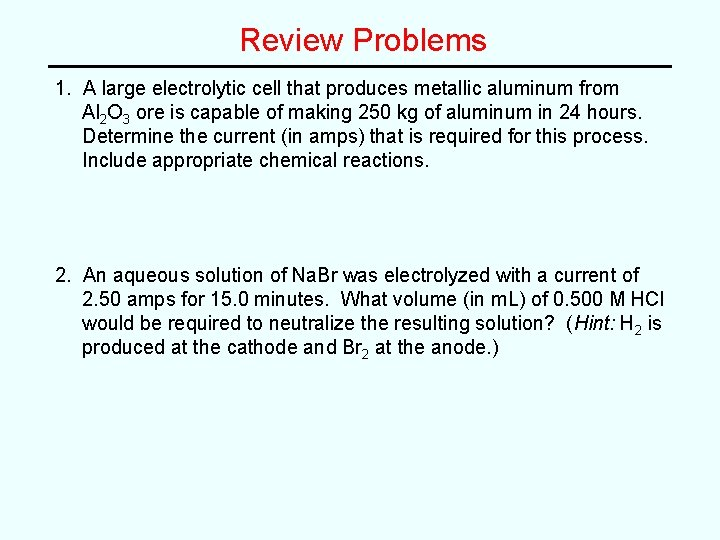Review Problems 1. A large electrolytic cell that produces metallic aluminum from Al 2 O 3 ore is capable of making 250 kg of aluminum in 24 hours. Determine the current (in amps) that is required for this process. Include appropriate chemical reactions. 2. An aqueous solution of Na. Br was electrolyzed with a current of 2. 50 amps for 15. 0 minutes. What volume (in m. L) of 0. 500 M HCl would be required to neutralize the resulting solution? (Hint: H 2 is produced at the cathode and Br 2 at the anode. )Review Problems 1. A large electrolytic cell that produces metallic aluminum from Al 2 O 3 ore is capable of making 250 kg of aluminum in 24 hours. Determine the current (in amps) that is required for this process. Include appropriate chemical reactions. 3. 1 x 104 amps (Al 3+(aq) + 3 e– --> Al(s) is ½-reaction, overall reaction is 2 Al 2 O 3(l) 3 O 2(g) + 4 Al(s)) 2. An aqueous solution of Na. Br was electrolyzed with a current of 2. 50 amps for 15. 0 minutes. What volume (in m. L) of 0. 500 M HCl would be required to neutralize the resulting solution? (Hint: H 2 is produced at the cathode and Br 2 at the anode. ) 46. 6 m. L HCl soln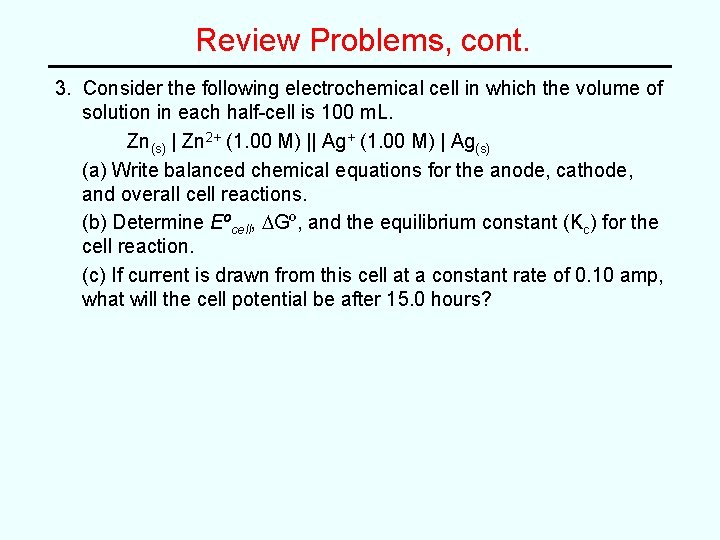Review Problems, cont. 3. Consider the following electrochemical cell in which the volume of solution in each half-cell is 100 m. L. Zn(s) | Zn 2+ (1. 00 M) || Ag+ (1. 00 M) | Ag(s) (a) Write balanced chemical equations for the anode, cathode, and overall cell reactions. (b) Determine Eºcell, DGº, and the equilibrium constant (Kc) for the cell reaction. (c) If current is drawn from this cell at a constant rate of 0. 10 amp, what will the cell potential be after 15. 0 hours?Answers 3. (a) Anode: Zn(s) --> Zn 2+(aq) + 2 e– Cathode: Ag+(aq) + e– --> Ag(s) Overall: Zn(s) + 2 Ag+(aq) --> Zn 2+(aq) + 2 Ag(s) (b) Eºcell = + 1. 56 V DG = - 301 k. J/mol Kc = 6 x 1052 (c) 1. 54 V